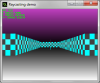# Raycasting engine - help needed

#### ras maxim

##### Member
Hello. I am trying to implement a simple raycasting algorythm in Game Maker Studio 1.4 following the tutorials by Permadi and Lode Vandevenne and I need some help.

Things I want to improve:

1. Division by zero bug. When checking for intersections with horizontal walls, we use the following formula (taken from here):
Code:
``first wall x coordinate = wall size / tangent of ray angle``
It works except the case when angle is 0 or 180 degrees. Because the tangent is zero, and division by zero returns infinity (-1.J, which is being rounded to zero by GMS, I guess) the x coordinate is always zero. It results in checking the wrong wall and displaying a pixel column that is totally out of place (see the screenshot, it is in the center).2. Correct floor drawing. I can't seem to find the right way to get the exact floor intersection coordinates. Lode Vandevenne gives the following code depending what wall is being cheked, east, west, south or north:
Code:
``````      //4 different wall directions possible
if(side == 0 && rayDirX > 0)
{
floorXWall = mapX;
floorYWall = mapY + wallX;
}
else if(side == 0 && rayDirX < 0)
{
floorXWall = mapX + 1.0;
floorYWall = mapY + wallX;
}
else if(side == 1 && rayDirY > 0)
{
floorXWall = mapX + wallX;
floorYWall = mapY;
}
else
{
floorXWall = mapX + wallX;
floorYWall = mapY + 1.0;
}``````
Where wallX and wallY are the exact coordinates of wall and ray intersection, mapX and mapY are the coorinates of the square where the wall is (coordinates divided by wall size and rounded) and floorXWall and floorYWall are exact coordinates of the ray intersection with the floor (I guess so).

It doesn't work for me, probably because I use pixel coordinates and lodev's tutorial uses grid coordinates (e.g. the first wall in the row is 0.0, 0.0 and the next one is 1.0, 0.0 and not 64.0, 0.0). For the current moment I just use wall coordinates for this, and it gives "scrolling floors" effect. This is not the way to go.

I can't figure out how to adapt these formulas for my pixel coordinates. If you know how to do it, please tell me.

3. Performance. It runs about 8 fps and 22 fps with YoYo Complier. I tried using surface buffers instead of draw_sprite, it frees another 3-4 frames. But the main time is taken by the floor drawing loop calculations. Even if I comment out the actual drawing part it is 14 fps and 36 fps with YoYo Complier.

If you have suggestions how to optimize the code, please post them.
Once again, you can find the link to the source code and the compiled executable at the top of the post. Thanks for reading.

•slojanko

#### ras maxim

##### Member
Haven't found a solution yet, so I think I can bump this thread.

#### ras maxim

##### Member``Xa = 64/Tan(alpha)``